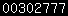In my 47 GHz EME setup I am using an LNA and a Kuhne transverter. The transverter uses an IF of 144 MHz which might cause problems in rejection of the image frequency. The transverter uses Image rejection mixer for suppressing the image frequency Looking at specifications of the transverter we see an image rejection of typical 17 dB but it could be worse. I tried to find out if I needed to use a filter between the LNA and the transverter. If we do not reject the image we will get a loss of 3 dB in system noise figure, the image frequency noise full adds to the noise of the wanted frequency and the effect is that noise power on the IF is 2 times as high as wanted and therefore loosing 3 dB in noise figure. Now it's not so difficult to calculate the loss in noise figure as function of the image rejection loss Putting the results in Excel I made this diagramAt 17 dB image rejection we will lose 0,1 dB in system noise figure